# Rational Function

Rational Function

Did you know Rational functions find application in different fields in our day-to-day life?

Not only do they describe the relationship between speed, distance, and time, but also are widely used in the medical and engineering industry

Once you understand the basics of rational functions, you will be able to plot a rational function graph, solve rational function problems, and even distinguish between the different types of rational functions.

Check out the interactive examples to know more about the lesson and try your hand at solving a few interesting practice questions at the end of the page.

## Lesson Plan

 1 What Are Rational Functions? 2 Important Notes on Rational Functions 3 Challenging Questions on Rational Functions 4 Solved Examples on Rational Functions 5 Interactive Questions on Rational Functions

## What Are Rational Functions?

A rational function is simply the ratio of polynomials.

Any function of one variable, x, is called a rational function if, it can be represented as the following rational function formula:

$\text {f(x) }= \dfrac { \text {p(x)}}{ \text {q(x) }}$

where $$\text p$$ and  $$\text q$$ are polynomial functions of  $$\text x$$ and  $$\text {q(x)} ≠ 0$$.

For example, $\text { f(x) } = \dfrac{ \text x^2+ \text x-2}{ \text x^2−2 \text x−3}$

is a rational function, where

${ \text x^2−2 \text x−3} ≠ 0$

## What is the General Form of a Rational Fraction?

The general form of a rational function is $\dfrac {\text {p(x)}}{\text {q(x)}}$

where $$\text {p(x)}$$ and $$\text { q(x) }$$ are polynomials and $$\text { q(x) } ≠ 0$$.

Examples:

$$\text y = \dfrac5{\text {x}} , \text { y} = \dfrac {3\text {x}+1}{\text {x}-4}, \text { y} = \dfrac2 { \text {x}^2 }, etc.$$

## How Does One Identify If a Function is Rational or Not?

In a rational function, there are a few operations that are not allowed to be used in the function.
Let us understand them by the following examples.

Example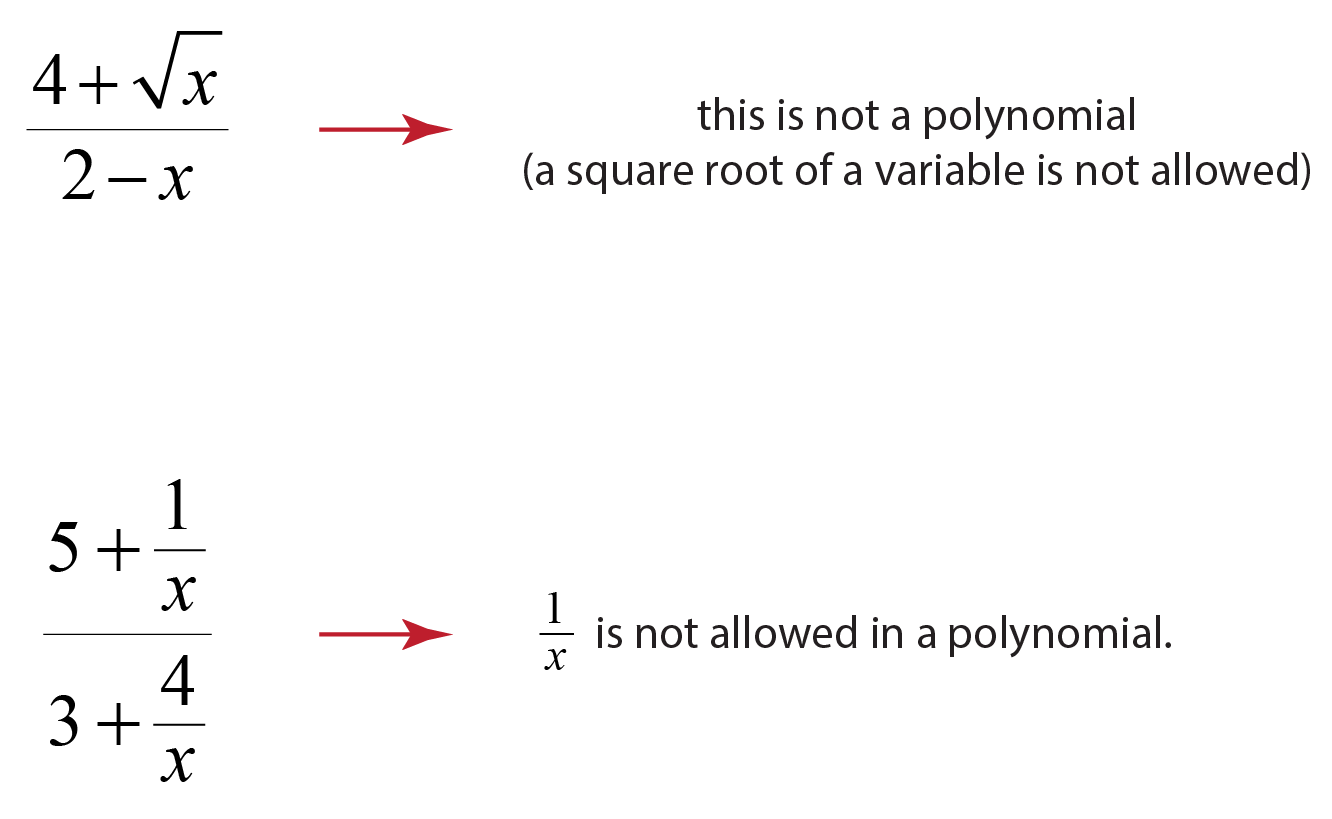## What Are Asymptotes?

• An asymptote is a line that the graph of the function approaches, but never touches.
• There are three types of asymptotes: horizontal, vertical, and oblique.
• Vertical asymptotes can be found out by finding the real zeros of the denominator.
• Horizontal asymptotes can be found out by thinking about the behavior of the function as $$\text { x }$$ approaches $$± { \infty}$$.

A rational function,

• Has horizontal asymptote when the degree of the numerator is less than or equal to the denominator
• Has oblique asymptote when the degree of the numerator is greater than that of the denominator.

For example:

A rational function in the form $\text {y} = \dfrac { \text a } { \text x- \text b} + \text c$ has a vertical asymptote at  $$\text {x = b}$$ , and a horizontal asymptote at $$\text { y = c }$$.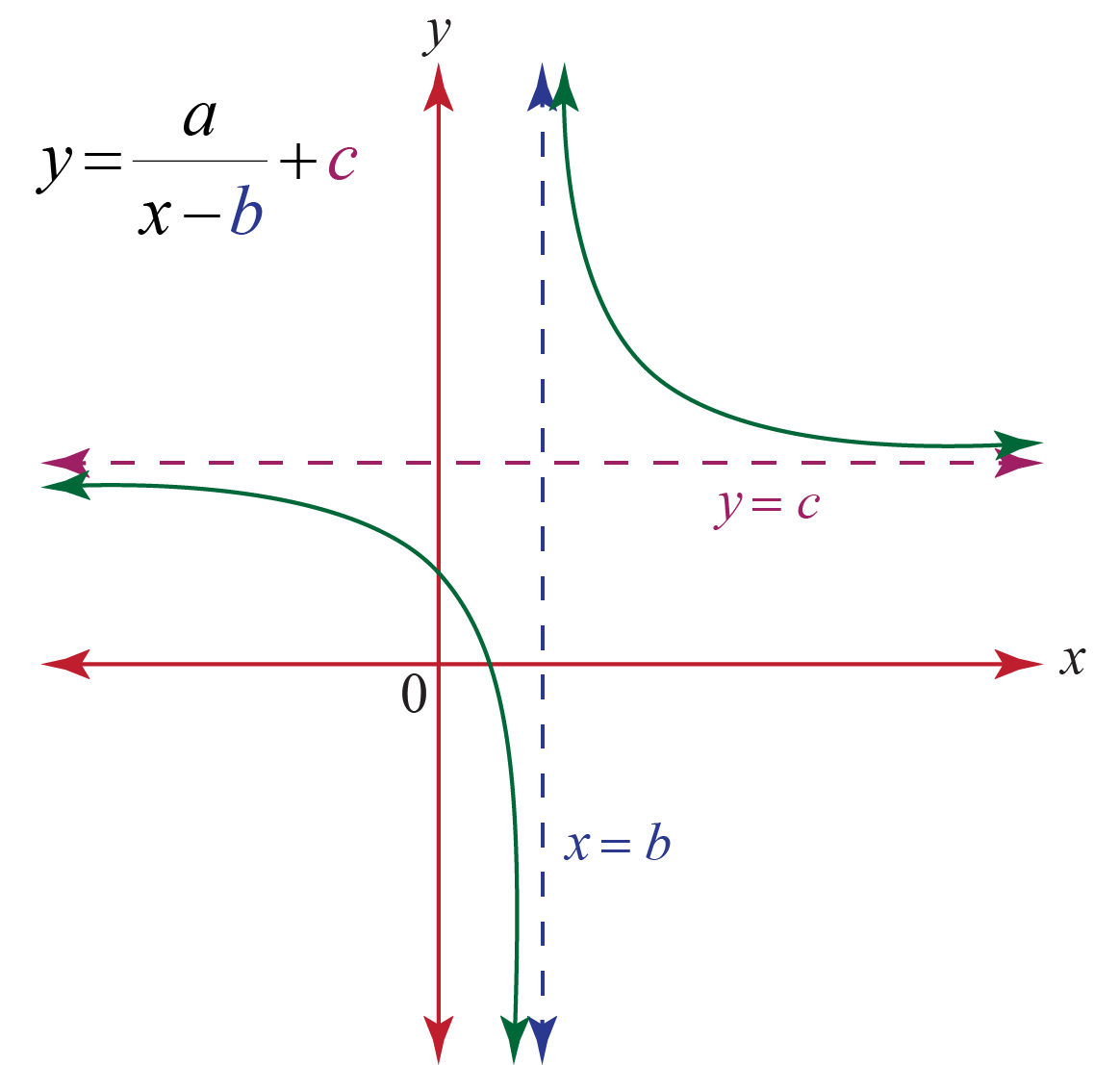## How to Plot a Rational Function Graph?

Procedure

Step1: Find the intercepts, if there are any.

Step2: Find the vertical asymptotes by setting the denominator equal to zero and solving.

Step3: Find the horizontal asymptote, if it exists, using the fact above.

Step4: Sketch the asymptote(s) and plot the y-intercept and any x-intercepts on your graph.

Step5: Sketch the graph.

Let us use the above steps to plot the graph for the parent function of a rational function in the next section.

### Graph for the Parent Function

The graph for the parent function, $$\text { f(x) } = \dfrac1 { \text {x }}$$ is a hyperbola .

Let's find the value of the function for different values of x.

x = -4, f(x) = -0.25

x = -2, f(x) = -0.5

x = -1, f(x) = -1

x = 1, f(x) = 1

x = 2, f(x) = 0.5

x = 4, f(x) = 0.25

So, as  $$\text {x }$$ get large the function keeps the sign of $$\text {x }$$ and gets smaller and smaller.

Similarly, as function approache $$\text {x } = 0$$, the function again keeps the same sign as $$\text {x }$$ but starts getting quite large.

Here is the sketch of this graph.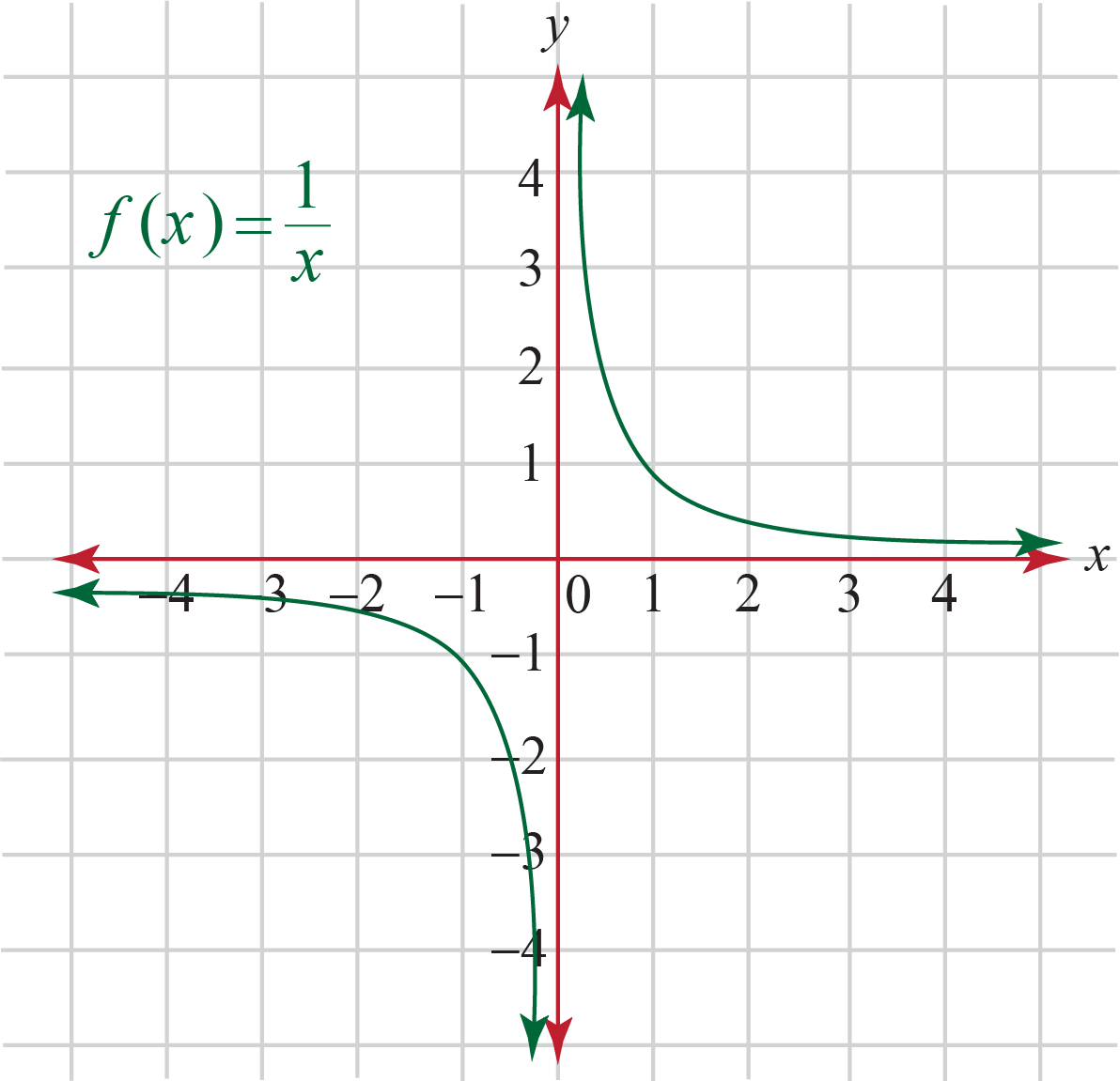### Domain

The domain and range is the set of all real numbers except 0.

Domain: $${ \text { x } | \text { x } ≠ 0}$$

Range:   $${ \text { y } | \text { y} ≠ 0}$$Challenging Questions

Find the formula for the function f (x), such that:

1. f (x) has a  horizontal asymptote at y = -5.
2. f (x) has vertical asymptotes at x = 2 and x = -2.
3. No x-intercept.

## Solved Examples

 Example 1

Find the x-intercepts of the function give below:

$\text { f(x) } = \dfrac{ \text x^2+ \text x-2}{ \text x^2−2 \text x−3}.$

Solution

Set the numerator of this rational function equal to zero and solve for $$\text {x}$$:

\begin{align*} \text x^2+ \text x - 2 &= 0 \\ ( \text x−1)( \text x+2) &= 0 \end{align*}

Solutions for this polynomial are -

$$\text x=1 \text { or } \text x=-2$$

 $$\therefore \text { x-intercepts at } 1 \text { and } -2$$
 Example 2

Find the roots of:

$$\text {g(x)} = \dfrac{3 \text x^3−6 \text x} { \text x^2−5}$$

and plot the graph for the function.

Solution

Factoring the numerator, we have

\begin{align*} 3 \text x^3−6 \text x &= 0 \\ 3 \text x( \text x^2−2) &= 0 \end{align*}

Given the factor $$3 \text x$$, the polynomial equals $$0$$ when $$3 \text x = 0$$ or $$\text x = 0$$.

Let the second factor equal zero, and solve for $$\text x$$ :

\begin{align*} \text x^2−2 &= 0 \\ \text x^2 &= 2 \\ \text x &= ±√2 \end{align*}

Thus, the three roots or x-intercepts are:

$0, −√2 \text { and } √2$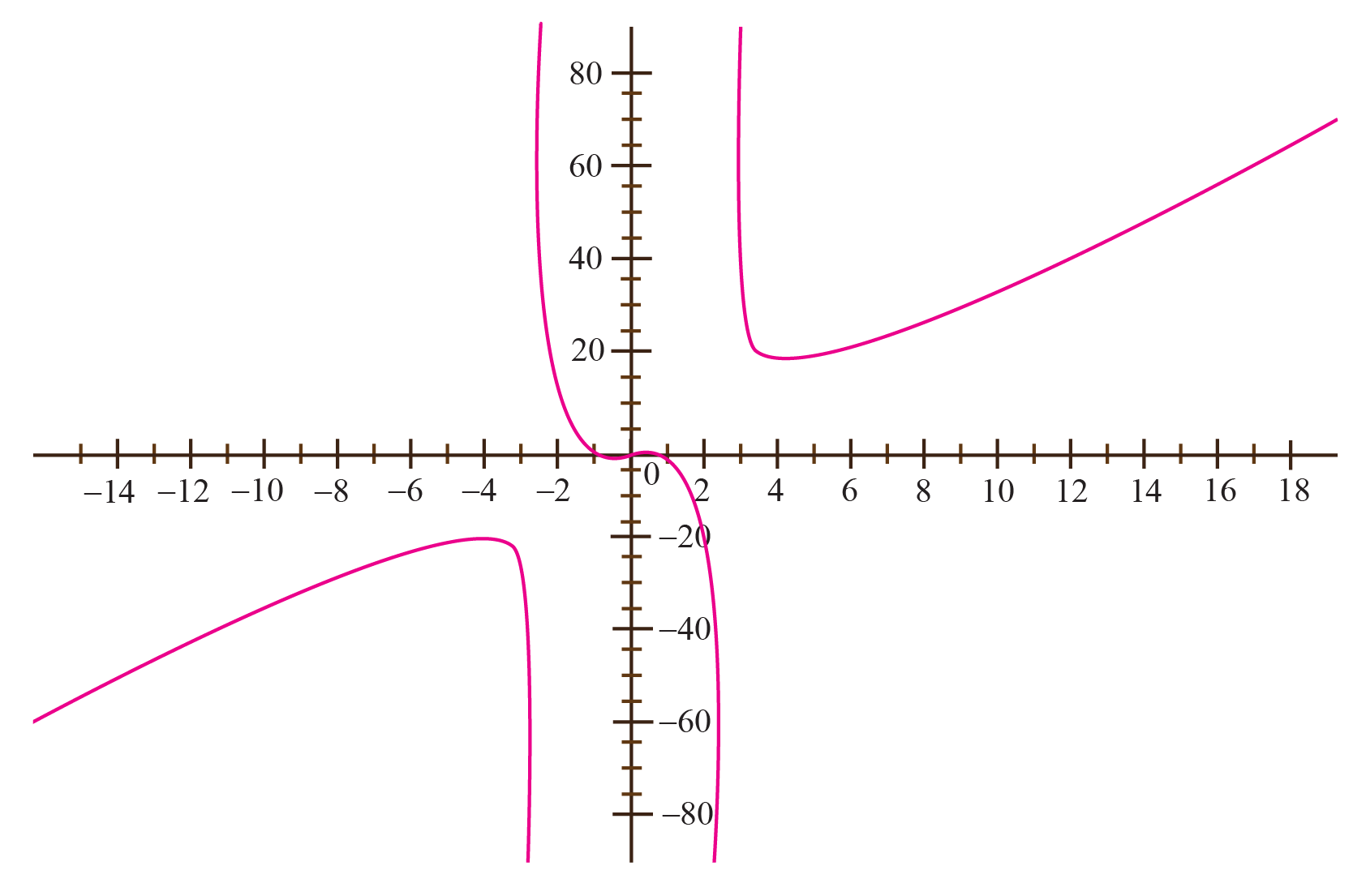$$\therefore \text{ x-intercepts }: 0, −√2 \text{ and } √2$$
 Example 3

Sketch the graph of the following function.
$\text { f(x) } = \dfrac { \text x+3 }{ \text x−1}$

Solution

So, we’ll start off with the intercepts.

The $$\text { y-intercept}$$ is,

$\text f(0)= \dfrac {0+3 }{0−1} = -3$

⇒ $\text {y-intercept }= (0,-3)$

The $$\text { x-intercepts }$$ will be,

\begin{align*} \text x+3 &= 0 \\ \text x &= −3 \end{align*}

⇒  $\text { x-intercepts } = (−3,0)$

Now, we need to determine the asymptotes.

Let’s first find the vertical asymptotes.

\begin{align*} \text x −1 &= 0 \\ \text x &= 1 \end{align*}
So, we’ve got one vertical asymptote. This means that there are now two regions of $$\text x$$’s.

They are $$\text x < 1$$ and $$\text x >1$$.

Now, the largest exponent in the numerator and denominator is $$1$$ and so by the fact, there will be a horizontal asymptote at the line.

$\text y=\dfrac11=1$

Now, we just need points in each region of $$\text x$$’s.

Since the $$\text {y-intercept }$$ and $$\text { x-intercept }$$ are already in the left region we won’t need to get any points there.

That means that we’ll just need to get a point in the right region.

It doesn’t really matter what value of $$\text x$$ we pick here we just need to keep it fairly small so it will fit onto our graph.

$\text f(2)=\dfrac{(2)+3} {2−1} = 5$

⇒ $$(2,5)$$
Okay, putting all this together gives the following graph.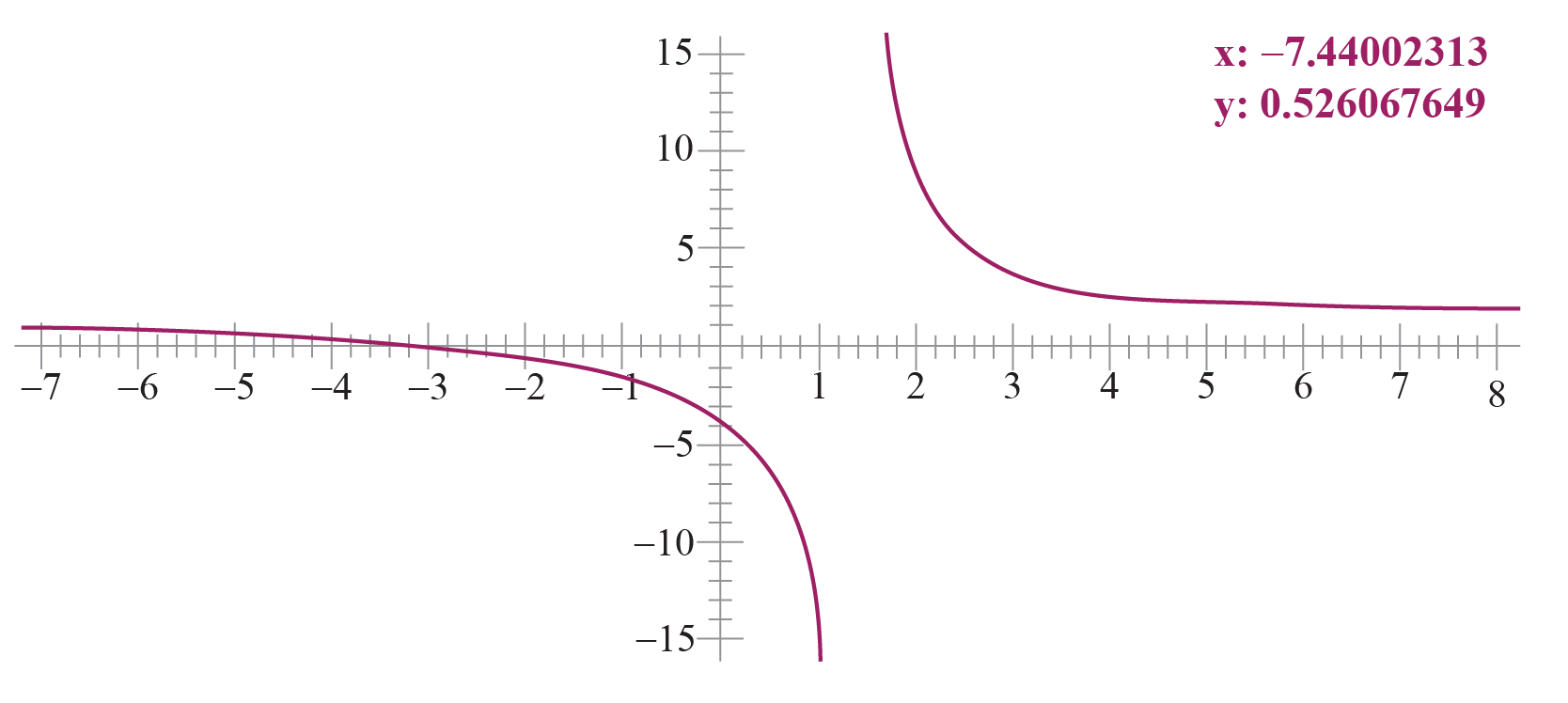Example 4

Find the vertical asymptotes of

$\text { f(x) } = \dfrac {(\text x+4)(x+3)}{( \text x+4)^2( \text x+1)}$

Solution

Notice that, based on the linear factors in the denominator, singularities exists at $$\text x= -4$$ and $$\text x= -1$$.

Also, notice that one linear factor $$( \text x+4)$$ cancels with the numerator.

However, one linear factor $$( \text x+4)$$ remains in the denominator because it is squared.

Therefore, a vertical asymptote exists at
$\text x= -4$
The linear factor $$( \text x+1)$$ also does not cancel out; thus, a vertical asymptote also exists at

$\text x = −1$

Vertical asymptotes are at

 $$\therefore$$ $$\text x = -1 \text { and } \text x = −4$$
 Example 5

Find any horizontal or oblique asymptote of

$\text { f(x)} = \dfrac {6 \text x^2 + \text x + 1}{ 3 \text x^2 + 16}$

Solution

Since, the polynomials in the numerator and denominator have the same degree $$(2)$$, we can identify that there is one horizontal asymptote and no oblique asymptote.

The coefficient of the highest power term is $$6$$ in the numerator and $$3$$ in the denominator.

Hence, horizontal asymptote is given by $$\text y= \dfrac63=2$$

 $$\therefore \text {horizontal asymptote} : \text y = 2$$

## Interactive Questions

Here are a few activities for you to practice.

## Let's Summarize

We hope you enjoyed learning about rational function with the simulations and practice questions. Now you will be able to easily solve problems on solving rational function, rational function graph, rational function formula, types of a rational function, and rational function calculator.

At Cuemath, our team of math experts is dedicated to making learning fun for our favorite readers, the students!

Through an interactive and engaging learning-teaching-learning approach, the teachers explore all angles of a topic.

Be it worksheets, online classes, doubt sessions, or any other form of relation, it’s the logical thinking and smart learning approach that we, at Cuemath, believe in.

## 1. What is the purpose of a rational function?

The purpose of a rational function is mainly in the field of numerical analysis. It is used for interpolation and approximation of functions.

Approximations using rational functions are well suited for computer algebra systems and other numerical software.

The wider applications of rational functions include approximation or model complex equations in science and engineering etc.

## 2. How can we use rational functions in real life?

Rational functions and equations can be used in many real-life situations. We can use them to describe speed-distance-time relationships and modeling work problems.

They can also be used in problems related to mixing two or more substances.

Rational functions are widely used in the medical industry as well as the engineering areas of study to help predict outcomes etc.

## 3. What are rational coefficients?

In any polynomial equation, the term before the variable is known as a coefficient.

A coefficient is a number multiplied by a variable.

For example,

$$2 \text x$$ in  this $$2$$ is  a coefficient.

Now any coefficient which can be written in form:

$$\text {f(x) }= \dfrac { \text {P(x)}}{ \text {Q(x) }}$$

are known rational coeffcient.

More Important Topics
Numbers
Algebra
Geometry
Measurement
Money
Data
Trigonometry
Calculus
More Important Topics
Numbers
Algebra
Geometry
Measurement
Money
Data
Trigonometry
Calculus
Learn from the best math teachers and top your exams

• Live one on one classroom and doubt clearing
• Practice worksheets in and after class for conceptual clarity
• Personalized curriculum to keep up with school